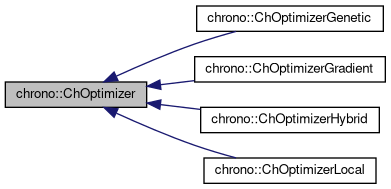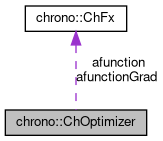chrono::ChOptimizer Class Reference

## Description

Base class for multi-variable optimization.

#include <ChSolvmin.h>

Inheritance diagram for chrono::ChOptimizer:[legend]
Collaboration diagram for chrono::ChOptimizer:[legend]

## Public Member Functions

ChOptimizer (const ChOptimizer &other)

virtual void SetObjective (ChFx *mformula)
Sets the objective function to maximize.

virtual ChFxGetObjective () const

Sets the objective function gradient (not mandatory, because if not set, the default bacward differentiation is used).

virtual void SetNumOfVars (int mv)
Set the number of optimization variables. More...

virtual int GetNumOfVars () const
Returns the number of optimization variables.

double * GetXv () const

double * GetXv_sup () const

double * GetXv_inf () const

void SetXv (double *mx)

void SetXv_sup (double *mx)

void SetXv_inf (double *mx)

double Eval_fx (double x[])
Returns the value of the functional, for given state of variables and with the given "database" multibody system. More...

double Eval_fx (const ChVectorDynamic<> &x)

void Eval_grad (double x[], double gr[])
Computes the gradient of objective function, for given state of variables. More...

void Eval_grad (const ChVectorDynamic<> &x, ChVectorDynamic<> &gr)

virtual bool PreOptimize ()
Performs the optimization of the ChSystem pointed by "database" (or whatever object which can evaluate the string "function" and the "optvarlist") using the current parameters. More...

virtual bool DoOptimize ()
This function computes the optimal xv[]. More...

virtual bool PostOptimize ()
Finalization and cleanup.

virtual bool Optimize ()
Does the three steps in sequence PreOptimize, DoOptimize, PostOptimize. More...

void DoBreakCheck ()
Each break_cycles number of times this fx is called, the function break_funct() is evaluated (if any) and if positive, the variable user_break becomes true.

## Public Attributes

bool minimize
default = false; just maximize

default = 1.e-12; step size for evaluation of gradient

double opt_fx
best resulting value of objective function

char err_message 
the ok/warning/error messages are written here

int error_code

long fx_evaluations
number of function evaluations

int(* break_funct )()
if not null, this function is called each 'break_cycles' evaluations

int break_cycles
how many fx evaluations per check

int user_break
if break_funct() reported true, this flag is ON, and optimizers should exit all cycles

int break_cyclecounter
internal

## Protected Attributes

ChFxafunction
the function to be maximized

the gradient of the function to be maximized, or null for default BDF.

int C_vars
number of input variables

double * xv
Vector of variables, also 1st approximation.

double * xv_sup
These are the hi/lo limits for the variables,.

double * xv_inf
these are not used by all optimizer, and can be NULL for gradient, but needed for genetic.

## ◆ DoOptimize()

 bool chrono::ChOptimizer::DoOptimize ( )
virtual

This function computes the optimal xv[].

It must be implemented by derived classes

## ◆ Eval_fx()

 double chrono::ChOptimizer::Eval_fx ( double x[] )

Returns the value of the functional, for given state of variables and with the given "database" multibody system.

Here evaluates the string "function".

 void chrono::ChOptimizer::Eval_grad ( double x[], double gr[] )

Computes the gradient of objective function, for given state of variables.

The gradient is stored into gr vector.

## ◆ Optimize()

 bool chrono::ChOptimizer::Optimize ( )
virtual

Does the three steps in sequence PreOptimize, DoOptimize, PostOptimize.

The derived classes shouldn't need the definition of this method, because they just have to implement the DoOptimize.

## ◆ PreOptimize()

 bool chrono::ChOptimizer::PreOptimize ( )
virtual

Performs the optimization of the ChSystem pointed by "database" (or whatever object which can evaluate the string "function" and the "optvarlist") using the current parameters.

Returns false if some error occurred. This function just makes some tests, allocations, and compilations..

## ◆ SetNumOfVars()

 virtual void chrono::ChOptimizer::SetNumOfVars ( int mv )
inlinevirtual

Set the number of optimization variables.

Note: this must be set properly as the number of variables used in the objective function!

Reimplemented in chrono::ChOptimizerHybrid.

The documentation for this class was generated from the following files:
• /builds/uwsbel/chrono/src/chrono/solver/ChSolvmin.h
• /builds/uwsbel/chrono/src/chrono/solver/ChSolvmin.cpp